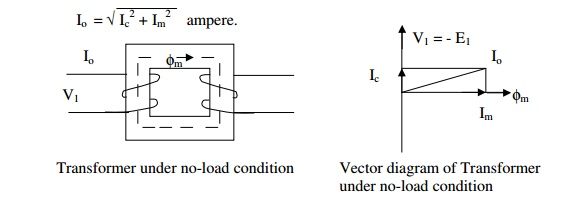Home | | Design of Electrical Machines | No-load current of a transformer

# No-load current of a transformer

The phasor sum of the magnetizing current (Im) and the loss component of current (I1) ; Im is calculated using the MMF/m required for the core and yoke and their respective length of flux path.

The phasor sum of the magnetizing current (Im) and the loss component of current (I1) ; Im is calculated using the MMF/m required for the core and yoke and their respective length of flux path. I1 is determined using the iron loss curve of the material used for the core and yoke and the flux density employed and their weight.

The no-load current I0 is the vectorial sum of the magnetizing current Im and core loss or working component current Ic. [Function of Im is to produce flux φm in the magnetic circuit and the function of Ic is to satisfy the no load losses of the transformer]. Thus,No load input to the transformer = V1I0Cosφ0 = V1Ic = No load losses as the output is zero and  input = output + losses.

Since the copper loss under no load condition is almost negligible, the no load losses can entirely be taken  as due to core loss only. Thus the core loss component of the no load current

Ic = core loss / V1for single phase transformerswith the assumption that the magnetizing current is sinusoidal (which is not true in practice)

The magnetic circuit of a transformer consists of both iron and air path. The iron path is due to legs and yokes and air path is due to the unavoidable joints created by the core composed of different shaped stampings. If all the joints are assumed to be equivalent to an air gap of lg , then the total ampere turns for the transformer magnetic circuit is equal to AT for iron + 800,000lgBm. Therefore,Note:

1. In case of a transformer of normal design, the no load current will generally be less than about 2% of the full load current.

2. No load power factor Cosφ0 = Ic/I0 and will be around 0.2.

3. Transformer copper losses:

a) The primary copper loss at no load is negligible as I0 is very less.

b) The secondary copper loss is zero at no load, as no current flows in the secondary winding at no  load.

4. Core or iron loss:

Total core loss = core loss in legs + core loss in yokes.

The core loss can be estimated at design stage by referring to graph of core loss/kg versus flux  density.

Core loss in leg = loss/kg in leg x weight of leg in kg

= loss / kg in leg x volume of the leg (AiHw) x density of steel or iron used

Core loss in yoke = loss/kg in Yoke x volume of yoke (Ay x mean length of the yoke) x density of iron  used

The density of iron or steel used for the transformer core lies between 7.55 to 7.8 grams/cc.

Study Material, Lecturing Notes, Assignment, Reference, Wiki description explanation, brief detail
Design of Electrical Machines : Transformers : No-load current of a transformer |